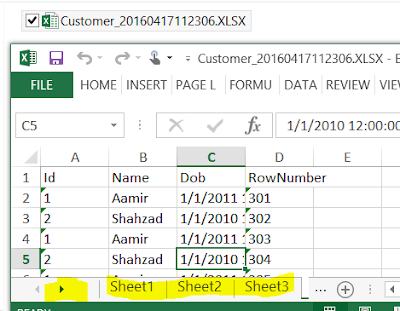### C# - How to Export large table data to multiple Excel Sheets on Single Excel file in C#

You are working as C# developer, You need to create a C# program that should read a large table from SQL Server database and export to multiple Excel Sheets on Single Excel file. You should be able to make decision how many records you would like to load to each Excel sheet.

The below C# script can be used to Export large table to multiple Excel sheets on Single Excel file. You don't have to worry about having unique column such as id etc. , As we generate row number dynamically for all the records and then divide them to multiple sheets.

```using System;
using System.Collections.Generic;
using System.Linq;
using System.Text;
using System.IO;
using System.Data.OleDb;
using System.Data.SqlClient;
using System.Data;

namespace TechBrothersIT.com_CSharp_Tutorial
{
class Program
{
static void Main(string[] args)
{

string datetime = DateTime.Now.ToString("yyyyMMddHHmmss");
string LogFolder = @"C:\Log\";
try
{

string FolderPath = @"C:\Destination\"; //Provide Destination folder path
string ExcelFileName = "Customer"; //Provide the Excel File Name you like to create
string TableName = "dbo.Customer";//Provide the table that you want to export to excel
Int32 RecordCntPerSheet = 100;//Provide the number of rows you want per sheet
string RecordCntPerSheetDecimal = RecordCntPerSheet + ".0";
ExcelFileName = ExcelFileName + "_" + datetime;

OleDbConnection Excel_OLE_Con = new OleDbConnection();
OleDbCommand Excel_OLE_Cmd = new OleDbCommand();

//Construct ConnectionString for Excel
string connstring = "Provider=Microsoft.ACE.OLEDB.12.0;" + "Data Source="
+ FolderPath + ExcelFileName
+ ";" + "Extended Properties=\"Excel 12.0 Xml;HDR=YES;\"";

//drop Excel file if exists
File.Delete(FolderPath + "\\" + ExcelFileName + ".xlsx");

//Create Connection to SQL Server
SqlConnection SQLConnection = new SqlConnection();
SQLConnection.ConnectionString = "Data Source = (local); Initial Catalog =TechBrothersIT; "
+ "Integrated Security=true;";

//Read distinct Group Values for each Excel Sheet
string query = "select ceiling(count(*)/" + RecordCntPerSheetDecimal + ") AS LoopCnt from " + TableName;
decimal LoopCnt = 0;

//Get the Count of Sheets need to be created
SqlCommand cmd = SQLConnection.CreateCommand();
cmd.CommandText = query;
SQLConnection.Open();
LoopCnt = (decimal)cmd.ExecuteScalar();
SQLConnection.Close();

string SheetName = "Sheet";
int startRowCnt = 0;
int endRowCnt = RecordCntPerSheet;

for (int sheetloop = 1; sheetloop <= LoopCnt; sheetloop++)
{
//Load Data into DataTable from SQL ServerTable
string queryString = ";with cte as (Select *, Row_Number() over (order by (Select 1)) AS RowNumber from " +
TableName + ") Select * From cte where RowNumber > " + startRowCnt.ToString() + " and RowNumber<=" + endRowCnt.ToString();
DataSet ds = new DataSet();

string TableColumns = "";

// Get the Column List from Data Table so can create Excel Sheet with Header
foreach (DataTable table in ds.Tables)
{
foreach (DataColumn column in table.Columns)
{
TableColumns += column + "],[";
}
}

// Replace most right comma from Columnlist
TableColumns = ("[" + TableColumns.Replace(",", " Text,").TrimEnd(','));
TableColumns = TableColumns.Remove(TableColumns.Length - 2);

//Use OLE DB Connection and Create Excel Sheet
Excel_OLE_Con.ConnectionString = connstring;
Excel_OLE_Con.Open();
Excel_OLE_Cmd.Connection = Excel_OLE_Con;
Excel_OLE_Cmd.CommandText = "Create table [" + SheetName
+ sheetloop.ToString() + "] ("
+ TableColumns + ")";
Excel_OLE_Cmd.ExecuteNonQuery();

//Write Data to Excel Sheet from DataTable dynamically
foreach (DataTable table in ds.Tables)
{
String sqlCommandInsert = "";
String sqlCommandValue = "";
foreach (DataColumn dataColumn in table.Columns)
{
sqlCommandValue += dataColumn + "],[";
}

sqlCommandValue = "[" + sqlCommandValue.TrimEnd(',');
sqlCommandValue = sqlCommandValue.Remove(sqlCommandValue.Length - 2);
sqlCommandInsert = "INSERT into [" + SheetName + sheetloop.ToString()
+ "] (" + sqlCommandValue + ") VALUES(";

int columnCount = table.Columns.Count;
foreach (DataRow row in table.Rows)
{
string columnvalues = "";
for (int i = 0; i < columnCount; i++)
{
int index = table.Rows.IndexOf(row);
columnvalues += "'" + table.Rows[index].ItemArray[i] + "',";

}
columnvalues = columnvalues.TrimEnd(',');
var command = sqlCommandInsert + columnvalues + ")";
Excel_OLE_Cmd.CommandText = command;
Excel_OLE_Cmd.ExecuteNonQuery();
}

}

startRowCnt += RecordCntPerSheet;
endRowCnt += RecordCntPerSheet;
Excel_OLE_Con.Close();
}

}
catch (Exception exception)
{
// Create Log File for Errors
using (StreamWriter sw = File.CreateText(LogFolder
+ "\\" + "ErrorLog_" + datetime + ".log"))
{
sw.WriteLine(exception.ToString());

}

}

}
}
}```

I executed the above script on for my dbo.Customer table and it was able to export 100 records on each sheet as I have set RecordsPerSheet=100 and export all the records to multiple excel sheets.How to export data to multiple excel sheets from single table by record count in C#

#### 1 comment:

1.I want to thank you since I was using COM library to create an excel file with 5000+ rows I realized that takes a while to complete that task but this approach it's better than mine, so thank you so much